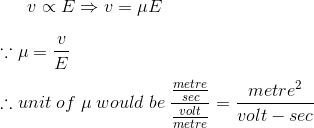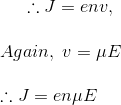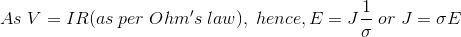# Mobility of Charge Carrier

This is a property of conductor, defined as the ratio of drift velocity to applied electric field in a conductor. Drift velocity of charge carriers in a conductor depend upon two factors, one is the intensity of applied electric field across the conductor and other is one property of the conductor called Mobility of Charge Carrier. In other words, for applied same electric field, on different metallic conductors there will be different drift velocities of electrons. These drift velocities of electrons depend upon a typical property of conductor called mobility of charge carrier.
In metal, the band occupied by the valance electrons may not be completely filled and hence there are no forbidden levels at higher energies. Because of that, there is always one or more free electrons available for many atoms which can freely move inside the metal. In metal that detached free electrons are not at all associated with their parent atoms and they move freely in the metal crystal by losing their individuality.

In other words no one electron can be said associated with any particular atom instead each free electron moves atom to atom in random manner. That means the metal can be visualized as a three-dimensional array of tightly bounded ions along with swarm of electrons move freely inside it. This conception is bitterly described as there is as such an electron gas inside a metal. According to the electron gas theory, electrons are in the metal in continuous motion, and the direction of the motion continuously being changed with each collision with heavy ions. The mean distance between two successive collisions is known as mean free path. As the directions of the motion of electrons inside the metal are completely randomized, there will be no resultant drifts of electrons in any particular direction in a given time hence the average current of the metal is zero in absence of any externally applied electric field.

Now let us assume that one electric field of Ε volt/metre is applied across the piece of metal. Due to influence of this electric field the free electrons will be accelerated. But due to collisions with much heavier ions, the velocity of electrons cannot be increased infinitely. At each collision the electron losses its kinetic energy and then regains its acceleration due to presence of external electric field. In this way the electrons reach to their finite steady Drift velocity after certain time of applied electric field. Let us assume this drift velocity is v metre/sec. It is needless to say the magnitude of this drift velocity of electrons is directly proportional to the intensity of the applied electric field Ε.Where, μ is the constant of proportionality and here it is called as mobility of electrons. This μ is generally known as Mobility of Charge Carrier and here the charge carriers are electrons. Now if the steady-state drift velocity is superimposed on the randomized thermal motion of the electrons, there will be a steady drift of electrons just in opposite to the direction of applied electric field.

This phenomenon constitutes an electric current. The current density J would be defined as, uniformly distributed current passing through a conductor per unit perpendicular cross-sectional area the conductor.
J = current density = current per unit area of conductor. More precisely current density can be defined as the uniformly distributed current passing through a conductor of unit cross-sectional area.
If the concentration of electrons per cubic metre is n,
nv = number of electrons crosses per unit time per unit cross-section of the conductor.
Therefore total charge crosses the unit cross-section of the conductor per unit time is env Coulombs. This is nothing but the current density of the conductor.Again for the conductor of unit dimension, cross-sectional area A = 1 m2, length L = 1 m, applied electric field E = V/L = V/1 = V (V is applied voltage across the conductor). Current I = J and resistance R = ρ = 1/σ, where, ρ is resistivity and σ is conductivity of the conductor.Want To Learn Faster? 🎓
Get electrical articles delivered to your inbox every week.
No credit card required—it’s 100% free.